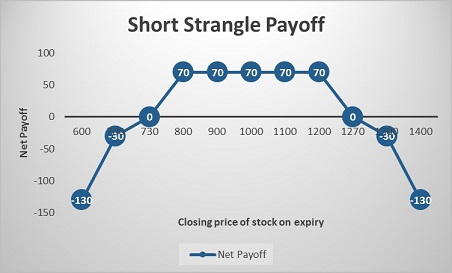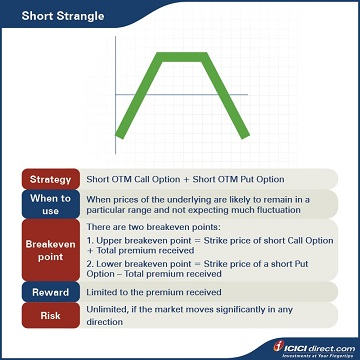Simran was impressed with Abhinav’s suggestions for PQR Ltd. She then asks him to think of other strategies that might work too. Here’s what Abhinav shares with her.

## Short Strangle

Abhinav suggests a Short Strangle as an alternative for PQR Ltd. A Short Strangle is a strategy that is similar to a Short Straddle, but is slightly less expensive.  The Options that a trader sells are normally OTM which are cheaper than ATM Options.

He suggests selling OTM Call and Put Options. This is in keeping with Simran’s view that prices of the underlying will remain in a particular range and not expecting much fluctuation.

With this strategy, you can experience unlimited loss with rewards capped up to the premium received.

Strategy: Short OTM Call Option (Leg 1) + Short OTM Put Option (Leg 2)

When to use: When prices of the underlying are likely to remain in a particular range and not expecting much fluctuation

Breakeven: There are two breakeven points:

1. Upper breakeven point = Strike price of short Call Option + Total premium received

2. Lower breakeven point = Strike price of a short Put Option – Total premium received

Maximum risk: Unlimited, if the market moves significantly in any direction

Let’s understand a Short Strangle strategy with an example:

Assume that the spot price of PQR Ltd. is Rs. 1,000. Abhinav sells a PQR Ltd. OTM Call of strike price Rs. 1,200 at Rs. 40 and Put Option of strike price Rs. 800 at Rs. 30. He receives total premium of Rs. 40 + Rs. 30 = Rs. 70 and this will be the maximum profit. However, he will accrue unlimited losses if the stock moves more than 70 points in either direction i.e., above 1200 + 70 = Rs. 1,270 or below 800 – 70 = Rs. 730.

### Let’s look at the cash flow in various scenarios:

 Closing price of stock on expiry (Rs.) Payoff from OTM Call Option (A) (Rs.) Payoff from OTM Put Option (B) (Rs.) Net payoff (A+B) (Rs.) 600 40 – 170 – 130 700 40 – 70 – 30 730 40 – 40 0 800 40 30 70 900 40 30 70 1000 40 30 70 1100 40 30 70 1200 40 30 70 1270 – 30 30 0 1300 – 60 30 – 30 1400 – 160 30 – 130

Let us understand the payoff in various scenarios. It will give you a fair idea of how we have arrived at the above values.

### If Stock closes at Rs. 700 on expiry: Leg 1 expires OTM while leg 2 expires ITM

Leg 1: Premium received on the OTM Call Option of strike price Rs. 1200 = Rs. 40

Premium paid on OTM Call Option of strike price Rs. 1200 at expiry = Max {0, (Spot price – Strike price)} = Max {0, (700 – 1200)} = Max (0, – 500) = 0

So, the payoff from the OTM Call Option = Premium received – Premium paid = 40 – 0 = Rs. 40

Leg 2: Premium received on the OTM Put Option of strike price Rs. 800 = Rs. 30

Premium paid on the OTM Put Option of strike price Rs. 800 at expiry = Max {0, (Strike price – Spot price)} = Max {0, (800 – 700)} = Max (0, 100) = Rs. 100

So, the payoff from the OTM Put Option = Premium received – Premium paid = 30 – 100 = – Rs. 70

Net payoff = Payoff from OTM Call Option + Payoff from OTM Put Option = 40 + (– 70) = – Rs. 30

### If the stock closes at 900 on expiry: Both the legs expire OTM

Leg 1: Premium received on the OTM Call Option of strike price Rs. 1200 = Rs. 40

Premium paid on the OTM Call Option of strike price Rs. 1200 at expiry = Max {0, (Spot price – Strike price)} = Max {0, (900 – 1200)} = Max (0, – 300) = 0

So, the payoff from the OTM Call Option = Premium received – Premium paid = 40 – 0 = Rs. 40

Leg 2: Premium received on the OTM Put Option of strike price Rs. 800 = Rs. 30

Premium paid on OTM Put Option of strike price Rs. 800 at expiry = Max {0, (Strike price – Spot price)} = Max {0, (800 – 900)} = Max (0, – 100) = 0

So, the payoff from the OTM Put Option = Premium received – Premium paid = 30 – 0 = Rs. 30

Net payoff = Payoff from OTM Call Option + Payoff from OTM Put Option = 40 + 30 = Rs. 70

### If the stock closes at 1270 on expiry: Leg 1 expires ITM while leg 2 expires OTM

Leg 1: Premium received on the OTM Call Option of strike price Rs. 1200 = Rs. 40

Premium paid on the OTM Call Option of strike price Rs. 1200 at expiry = Max {0, (Spot price – Strike price)} = Max {0, (1270 – 1200)} = Max (0, 70) = Rs. 70

So, the payoff from the OTM Call Option = Premium received – Premium paid = 40 – 70 = – Rs. 30

Leg 2: Premium received on the OTM Put Option of strike price Rs. 800 = Rs. 30

Premium paid on OTM Put Option of strike price Rs. 800 at expiry = Max {0, (Strike price– Spot price)} = Max {0, (800 – 1270)} = Max (0, – 470) = 0

So, the payoff from the OTM Put Option = Premium received – Premium paid = 30 – 0 = Rs. 30

Net payoff = Payoff from OTM Call Option + Payoff from OTM Put Option = (– 30) + 30 = 0## Summary• A Short Strangle is a two-part Options strategy that involves one short Call with a higher strike price and one short Put with a lower strike price. They are both on the same underlying, with the same expiration date, but different strike prices.
• Breakeven: There are two breakeven points:
• Upper breakeven point = Strike price of short Call Option + Total premium received
• Lower breakeven point = Strike price of a short Put Option – Total premium received